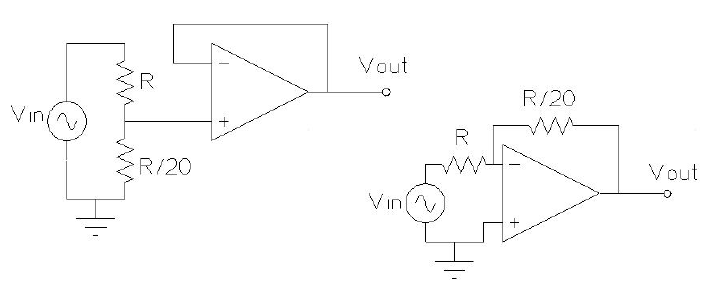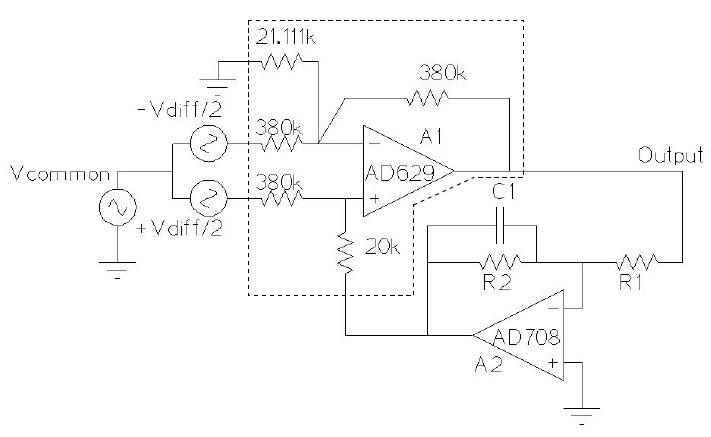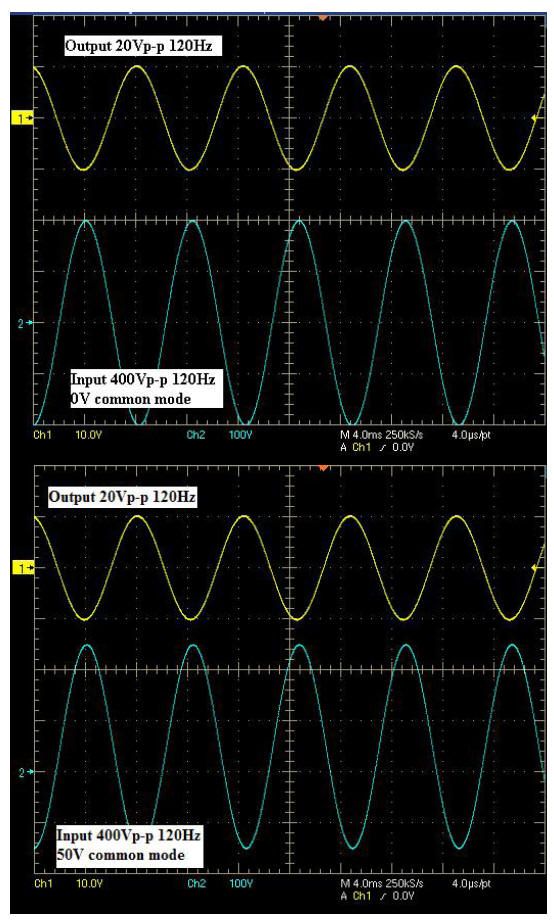# Adjustable-Gain Difference Amplifier Circuit Measures Hundreds of Volts, Rejects Large Common-Mode Signals

A high common-mode difference amplifier, in a feedback loop with an inverting op amp, is a useful aid for performing high-voltage differential measurements up to 500 V.

Two common solutions to monitor power line voltages or other large signals—using low-voltage electronics—involve a high-resistance voltage divider to attenuate the input, followed by an op amp buffer (Figure 1a); or an inverting op amp with high input resistance (Figure 1b). These methods have several shortcomings: they are useful mainly for single-ended measurements, making them prone to ground noise; they cannot reject common-mode voltages; and the resistors dissipate different amounts of power, leading to gain errors.Figure 1. Single-ended measurements using op amps.

A better way involves the use of a difference amplifier. The AD629 unity-gain diff amp, shown in Figure 2, can reject extremely high common-mode signals (up to 270 V with 15-V supplies). To achieve this high common-mode voltage range, a precision internal resistor divider attenuates the non-inverting signal by a factor of 20. Other internal resistors restore the gain to provide a differential gain of unity. The complete transfer function, with reference inputs grounded, is

Laser wafer trimming provides resistor matching for common-mode rejection (CMR) of up to 86 dB minimum.Figure 2. Functional block diagram of the AD629 difference amplifier.

A highly useful application, shown in Figure 3, uses the AD629 and an op amp, such as the AD708, to provide adjustable gain. A differential input signal is applied to difference amplifier A1, which rejects any common-mode voltage on the input. The differential input signal appears at the output with a gain of 1. The output voltage is fed into the input of operational amplifier A2, which is configured as a voltage inverter with a gain of –R2/R1. The inverter’s output voltage is applied to the difference amplifier’s reference pin (Ref+). The voltage applied to this pin is multiplied by a gain of 19, and is added to the output of A1. Solving for the output of this negative feedback loop,

Capacitor C1 (100 nF), with resistor R2 (20 kΩ), provides loop stability and sets the bandwidth at approximately 1 kHz.Figure 3. Difference amplifier in adjustable-gain configuration.

The maximum differential signal that the circuit can handle is limited by the output range of amplifiers A1 and A2. When R2/R1 is less than or equal to 1, amplifier A1’s output will saturate first. With ±15-V supplies, the circuit can handle differential voltages up to 520 Vpk-pk. Systems with ±10-V or ±5-V supply voltages can handle differential voltages up to 320 Vpk-pk and 120 Vpk-pk, respectively. With ratio R2/R1 greater than 1 and an amplifier with rail-to-rail output for A2, these ranges can be extended closer to 600 Vpk-pk, 400 Vpk-pk and 200 Vpk-pk.

Negative feedback around the loop helps to reduce the voltage seen by the inputs of op amp A1. This means that the circuit has the input range to reject large common-mode voltages, even in the presence of large differential voltages.

Figure 4 shows two plots: in the first plot, a differential signal of 400 Vpk-pk is measured using ±15 V supplies; in the second plot, a 400-Vpk-pk differential signal is measured in the presence of a 50-V common-mode voltage. As can be seen, this circuit allows very large differential inputs; rejects common-mode voltages; and allows the differential gain to be set by the ratio of resistors R2 and R1, enabling the user to select the appropriate level of attenuation. The resistors inside the difference amplifier are precision laser-trimmed and manufactured with low-drift thin film material, so the system does not suffer from resistor self-heating errors.Figure 4. 400-Vpk-pk differential signal measured with 0-V and 50-V common-mode signal.